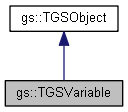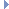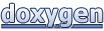# GameShield V5 SDK Programming Guide: gs::TGSVariable Class Reference

#### GameShield v5 SDK

 GameShield V5 SDK Programming Guide  v5.2
gs::TGSVariable Class Reference

User defined variables or parameters of action / licenses. More...

Inheritance diagram for gs::TGSVariable:[legend]
Collaboration diagram for gs::TGSVariable:[legend]

## Public Member Functions

template<>
void get (int64_t &v)
gets value as 64-bit integer

template<>
void get (float &v)
gets value as float

template<>
void get (double &v)
gets value as double

template<>
void set (int v)
sets value from 32-bit integer

template<>
void set (int64_t v)
sets value from 64-bit integer

template<>
void set (float v)
sets value from float

template<>
void set (double v)
sets value from double

Value Accessor
void fromString (const char *v)
set value from a string

void fromInt (int v)
set value from a 32-bit integerva

void fromBool (bool v)
set value from a boolean

void fromInt64 (int64_t v)
set value from a 64-bit integer

void fromFloat (float v)
set value from a float

void fromDouble (double v)
set value from a double

void fromUTCTime (time_t t)
set value from a time_t (total seconds since 01/01/1970)

const char * asString ()
get value as string

int asInt ()
get valie as 32-bit integer

bool asBool ()
get value as boolean

int64_t asInt64 ()
get value as 64-bit integer

float asFloat ()
get value as float

double asDouble ()
get value as double

time_t asUTCTime ()
get value as a time_t (total seconds since 01/01/1970)

Properties
const char * name ()
get the variable name

var_type_t typeId ()
get the variable type id. (ref: varType)

std::string attribute ()
get the variable attribute (ref: varAttr)

C++ template based setter/getter [ C++ Only ]
template<>
void get (int &v)
gets value as 32-bit integer

template<>
void get (int64_t &v)
gets value as 64-bit integer

template<>
void get (float &v)
gets value as float

template<>
void get (double &v)
gets value as double

template<>
void get (std::string &v)
gets value as string

template<>
void set (int v)
sets value from 32-bit integer

template<>
void set (int64_t v)
sets value from 64-bit integer

template<>
void set (float v)
sets value from float

template<>
void set (double v)
sets value from double

template<>
void set (std::string v)
sets value from stringPublic Member Functions inherited from gs::TGSObject
gs_handle_t handle () const
Gets GS5 object handle.

## Static Public Member Functions

static const char * getTypeName (var_type_t varType)
Converts variable type id to its type string. More...

Attribute conversion helpers
static int AttrFromString (const char *permitStr)
Get attribute value from its string representation. (Ref: varAttr)

static std::string AttrToString (int permit)
Get string representation from its attribute value. (Ref: varAttr)

## Detailed Description

User defined variables or parameters of action / licenses.

## Member Function Documentation

 const char * gs::TGSVariable::getTypeName ( var_type_t varType )
static

Converts variable type id to its type string.

Ref: varType

Generated on Mon Feb 3 2014 13:15:29 for GameShield V5 SDK Programming Guide by1.8.5